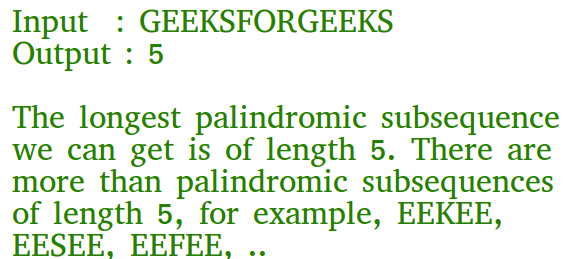C Program for Longest Palindromic Subsequence | DP-12

• Last Updated : 13 Aug, 2021

Given a sequence, find the length of the longest palindromic subsequence in it.As another example, if the given sequence is “BBABCBCAB”, then the output should be 7 as “BABCBAB” is the longest palindromic subsequence in it. “BBBBB” and “BBCBB” are also palindromic subsequences of the given sequence, but not the longest ones.

1) Optimal Substructure:
Let X[0..n-1] be the input sequence of length n and L(0, n-1) be the length of the longest palindromic subsequence of X[0..n-1].
If last and first characters of X are same, then L(0, n-1) = L(1, n-2) + 2.
Else L(0, n-1) = MAX (L(1, n-1), L(0, n-2)).

Following is a general recursive solution with all cases handled.

C

 // C program of above approach#include #include  // A utility function to get max of two integersint max(int x, int y) { return (x > y) ? x : y; } // Returns the length of the longest palindromic subsequence in seqint lps(char* seq, int i, int j){    // Base Case 1: If there is only 1 character    if (i == j)        return 1;     // Base Case 2: If there are only 2 characters and both are same    if (seq[i] == seq[j] && i + 1 == j)        return 2;     // If the first and last characters match    if (seq[i] == seq[j])        return lps(seq, i + 1, j - 1) + 2;     // If the first and last characters do not match    return max(lps(seq, i, j - 1), lps(seq, i + 1, j));} /* Driver program to test above functions */int main(){    char seq[] = "GEEKSFORGEEKS";    int n = strlen(seq);    printf("The length of the LPS is %d", lps(seq, 0, n - 1));    getchar();    return 0;}
Output:
The length of the LPS is 5

Dynamic Programming Solution

C

 // A Dynamic Programming based C program for LPS problem// Returns the length of the longest palindromic subsequence in seq#include #include  // A utility function to get max of two integersint max(int x, int y) { return (x > y) ? x : y; } // Returns the length of the longest palindromic subsequence in seqint lps(char* str){    int n = strlen(str);    int i, j, cl;    int L[n][n]; // Create a table to store results of subproblems     // Strings of length 1 are palindrome of length 1    for (i = 0; i < n; i++)        L[i][i] = 1;     // Build the table. Note that the lower diagonal values of table are    // useless and not filled in the process. The values are filled in a    // manner similar to Matrix Chain Multiplication DP solution (See    // https:// www.geeksforgeeks.org/matrix-chain-multiplication-dp-8/). cl is length of    // substring    for (cl = 2; cl <= n; cl++) {        for (i = 0; i < n - cl + 1; i++) {            j = i + cl - 1;            if (str[i] == str[j] && cl == 2)                L[i][j] = 2;            else if (str[i] == str[j])                L[i][j] = L[i + 1][j - 1] + 2;            else                L[i][j] = max(L[i][j - 1], L[i + 1][j]);        }    }     return L[n - 1];} /* Driver program to test above functions */int main(){    char seq[] = "GEEKS FOR GEEKS";    int n = strlen(seq);    printf("The length of the LPS is %d", lps(seq));    getchar();    return 0;}
Output
The length of the LPS is 7

Please refer complete article on Longest Palindromic Subsequence | DP-12 for more details!

My Personal Notes arrow_drop_up